# A Problem that Stumped Milton Friedman¶

(and that Abraham Wald solved by inventing sequential analysis)

## Contents¶

In addition to what’s in Anaconda, this lecture will need the following libraries:

In :
!pip install quantecon
!pip install interpolation


## Overview¶

This lecture describes a statistical decision problem encountered by Milton Friedman and W. Allen Wallis during World War II when they were analysts at the U.S. Government’s Statistical Research Group at Columbia University.

This problem led Abraham Wald [Wal47] to formulate sequential analysis, an approach to statistical decision problems intimately related to dynamic programming.

In this lecture, we apply dynamic programming algorithms to Friedman and Wallis and Wald’s problem.

Key ideas in play will be:

• Bayes’ Law
• Dynamic programming
• Type I and type II statistical errors
• a type I error occurs when you reject a null hypothesis that is true
• a type II error is when you accept a null hypothesis that is false
• Abraham Wald’s sequential probability ratio test
• The power of a statistical test
• The critical region of a statistical test
• A uniformly most powerful test

We’ll begin with some imports:

In :
import numpy as np
import matplotlib.pyplot as plt
from numba import jit, prange, float64, int64
from numba.experimental import jitclass
from interpolation import interp
from math import gamma


This lecture uses ideas studied in this lecture, this lecture. and this lecture.

## Origin of the Problem¶

On pages 137-139 of his 1998 book Two Lucky People with Rose Friedman [FF98], Milton Friedman described a problem presented to him and Allen Wallis during World War II, when they worked at the US Government’s Statistical Research Group at Columbia University.

Let’s listen to Milton Friedman tell us what happened

In order to understand the story, it is necessary to have an idea of a simple statistical problem, and of the standard procedure for dealing with it. The actual problem out of which sequential analysis grew will serve. The Navy has two alternative designs (say A and B) for a projectile. It wants to determine which is superior. To do so it undertakes a series of paired firings. On each round, it assigns the value 1 or 0 to A accordingly as its performance is superior or inferior to that of B and conversely 0 or 1 to B. The Navy asks the statistician how to conduct the test and how to analyze the results.

The standard statistical answer was to specify a number of firings (say 1,000) and a pair of percentages (e.g., 53% and 47%) and tell the client that if A receives a 1 in more than 53% of the firings, it can be regarded as superior; if it receives a 1 in fewer than 47%, B can be regarded as superior; if the percentage is between 47% and 53%, neither can be so regarded.

When Allen Wallis was discussing such a problem with (Navy) Captain Garret L. Schyler, the captain objected that such a test, to quote from Allen’s account, may prove wasteful. If a wise and seasoned ordnance officer like Schyler were on the premises, he would see after the first few thousand or even few hundred [rounds] that the experiment need not be completed either because the new method is obviously inferior or because it is obviously superior beyond what was hoped for $\ldots$.

Friedman and Wallis struggled with the problem but, after realizing that they were not able to solve it, described the problem to Abraham Wald.

That started Wald on the path that led him to Sequential Analysis [Wal47].

We’ll formulate the problem using dynamic programming.

## A Dynamic Programming Approach¶

The following presentation of the problem closely follows Dmitri Berskekas’s treatment in Dynamic Programming and Stochastic Control [Ber75].

A decision-maker observes a sequence of draws of a random variable $z$.

He (or she) wants to know which of two probability distributions $f_0$ or $f_1$ governs $z$.

Conditional on knowing that successive observations are drawn from distribution $f_0$, the sequence of random variables is independently and identically distributed (IID).

Conditional on knowing that successive observations are drawn from distribution $f_1$, the sequence of random variables is also independently and identically distributed (IID).

But the observer does not know which of the two distributions generated the sequence.

For reasons explained in Exchangeability and Bayesian Updating, this means that the sequence is not IID and that the observer has something to learn, even though he knows both $f_0$ and $f_1$.

The decision maker chooses a number of draws (i.e., random samples from the unknown distribution) and uses them to decide which of the two distributions is generating outcomes.

He starts with prior

$$\pi_{-1} = \mathbb P \{ f = f_0 \mid \textrm{ no observations} \} \in (0, 1)$$

After observing $k+1$ observations $z_k, z_{k-1}, \ldots, z_0$, he updates this value to

$$\pi_k = \mathbb P \{ f = f_0 \mid z_k, z_{k-1}, \ldots, z_0 \}$$

which is calculated recursively by applying Bayes’ law:

$$\pi_{k+1} = \frac{ \pi_k f_0(z_{k+1})}{ \pi_k f_0(z_{k+1}) + (1-\pi_k) f_1 (z_{k+1}) }, \quad k = -1, 0, 1, \ldots$$

After observing $z_k, z_{k-1}, \ldots, z_0$, the decision-maker believes that $z_{k+1}$ has probability distribution

$$f_{{\pi}_k} (v) = \pi_k f_0(v) + (1-\pi_k) f_1 (v) ,$$

which is a mixture of distributions $f_0$ and $f_1$, with the weight on $f_0$ being the posterior probability that $f = f_0$ .

To illustrate such a distribution, let’s inspect some mixtures of beta distributions.

The density of a beta probability distribution with parameters $a$ and $b$ is

$$f(z; a, b) = \frac{\Gamma(a+b) z^{a-1} (1-z)^{b-1}}{\Gamma(a) \Gamma(b)} \quad \text{where} \quad \Gamma(t) := \int_{0}^{\infty} x^{t-1} e^{-x} dx$$

The next figure shows two beta distributions in the top panel.

The bottom panel presents mixtures of these distributions, with various mixing probabilities $\pi_k$

In :
@jit(nopython=True)
def p(x, a, b):
r = gamma(a + b) / (gamma(a) * gamma(b))
return r * x**(a-1) * (1 - x)**(b-1)

f0 = lambda x: p(x, 1, 1)
f1 = lambda x: p(x, 9, 9)
grid = np.linspace(0, 1, 50)

fig, axes = plt.subplots(2, figsize=(10, 8))

axes.set_title("Original Distributions")
axes.plot(grid, f0(grid), lw=2, label="$f_0$")
axes.plot(grid, f1(grid), lw=2, label="$f_1$")

axes.set_title("Mixtures")
for π in 0.25, 0.5, 0.75:
y = π * f0(grid) + (1 - π) * f1(grid)
axes.plot(y, lw=2, label=f"$\pi_k$ = {π}")

for ax in axes:
ax.legend()
ax.set(xlabel="$z$ values", ylabel="probability of $z_k$")

plt.tight_layout()
plt.show()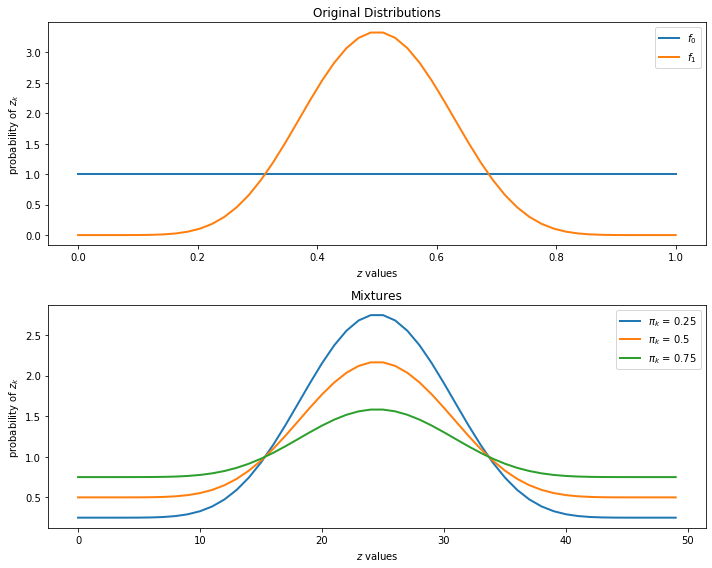### Losses and Costs¶

After observing $z_k, z_{k-1}, \ldots, z_0$, the decision-maker chooses among three distinct actions:

• He decides that $f = f_0$ and draws no more $z$’s
• He decides that $f = f_1$ and draws no more $z$’s
• He postpones deciding now and instead chooses to draw a $z_{k+1}$

Associated with these three actions, the decision-maker can suffer three kinds of losses:

• A loss $L_0$ if he decides $f = f_0$ when actually $f=f_1$
• A loss $L_1$ if he decides $f = f_1$ when actually $f=f_0$
• A cost $c$ if he postpones deciding and chooses instead to draw another $z$

### Digression on Type I and Type II Errors¶

If we regard $f=f_0$ as a null hypothesis and $f=f_1$ as an alternative hypothesis, then $L_1$ and $L_0$ are losses associated with two types of statistical errors

• a type I error is an incorrect rejection of a true null hypothesis (a “false positive”)
• a type II error is a failure to reject a false null hypothesis (a “false negative”)

So when we treat $f=f_0$ as the null hypothesis

• We can think of $L_1$ as the loss associated with a type I error.
• We can think of $L_0$ as the loss associated with a type II error.

### Intuition¶

Let’s try to guess what an optimal decision rule might look like before we go further.

Suppose at some given point in time that $\pi$ is close to 1.

Then our prior beliefs and the evidence so far point strongly to $f = f_0$.

If, on the other hand, $\pi$ is close to 0, then $f = f_1$ is strongly favored.

Finally, if $\pi$ is in the middle of the interval $[0, 1]$, then we have little information in either direction.

This reasoning suggests a decision rule such as the one shown in the figureAs we’ll see, this is indeed the correct form of the decision rule.

The key problem is to determine the threshold values $\alpha, \beta$, which will depend on the parameters listed above.

You might like to pause at this point and try to predict the impact of a parameter such as $c$ or $L_0$ on $\alpha$ or $\beta$.

### A Bellman Equation¶

Let $J(\pi)$ be the total loss for a decision-maker with current belief $\pi$ who chooses optimally.

With some thought, you will agree that $J$ should satisfy the Bellman equation

$$J(\pi) = \min \left\{ (1-\pi) L_0, \; \pi L_1, \; c + \mathbb E [ J (\pi') ] \right\} \tag{1}$$

where $\pi'$ is the random variable defined by Bayes’ Law

$$\pi' = \kappa(z', \pi) = \frac{ \pi f_0(z')}{ \pi f_0(z') + (1-\pi) f_1 (z') }$$

when $\pi$ is fixed and $z'$ is drawn from the current best guess, which is the distribution $f$ defined by

$$f_{\pi}(v) = \pi f_0(v) + (1-\pi) f_1 (v)$$

In the Bellman equation, minimization is over three actions:

1. Accept the hypothesis that $f = f_0$
2. Accept the hypothesis that $f = f_1$
3. Postpone deciding and draw again

We can represent the Bellman equation as

$$J(\pi) = \min \left\{ (1-\pi) L_0, \; \pi L_1, \; h(\pi) \right\} \tag{2}$$

where $\pi \in [0,1]$ and

• $(1-\pi) L_0$ is the expected loss associated with accepting $f_0$ (i.e., the cost of making a type II error).
• $\pi L_1$ is the expected loss associated with accepting $f_1$ (i.e., the cost of making a type I error).
• $h(\pi) := c + \mathbb E [J(\pi')]$ the continuation value; i.e., the expected cost associated with drawing one more $z$.

The optimal decision rule is characterized by two numbers $\alpha, \beta \in (0,1) \times (0,1)$ that satisfy

$$(1- \pi) L_0 < \min \{ \pi L_1, c + \mathbb E [J(\pi')] \} \textrm { if } \pi \geq \alpha$$

and

$$\pi L_1 < \min \{ (1-\pi) L_0, c + \mathbb E [J(\pi')] \} \textrm { if } \pi \leq \beta$$

The optimal decision rule is then

\begin{aligned} \textrm { accept } f=f_0 \textrm{ if } \pi \geq \alpha \\ \textrm { accept } f=f_1 \textrm{ if } \pi \leq \beta \\ \textrm { draw another } z \textrm{ if } \beta \leq \pi \leq \alpha \end{aligned}

Our aim is to compute the value function $J$, and from it the associated cutoffs $\alpha$ and $\beta$.

To make our computations simpler, using (2), we can write the continuation value $h(\pi)$ as

\begin{aligned} h(\pi) &= c + \mathbb E [J(\pi')] \\ &= c + \mathbb E_{\pi'} \min \{ (1 - \pi') L_0, \pi' L_1, h(\pi') \} \\ &= c + \int \min \{ (1 - \kappa(z', \pi) ) L_0, \kappa(z', \pi) L_1, h(\kappa(z', \pi) ) \} f_\pi (z') dz' \end{aligned} \tag{3}

The equality

$$h(\pi) = c + \int \min \{ (1 - \kappa(z', \pi) ) L_0, \kappa(z', \pi) L_1, h(\kappa(z', \pi) ) \} f_\pi (z') dz' \tag{4}$$

can be understood as a functional equation, where $h$ is the unknown.

Using the functional equation, (4), for the continuation value, we can back out optimal choices using the right side of (2).

This functional equation can be solved by taking an initial guess and iterating to find a fixed point.

Thus, we iterate with an operator $Q$, where

$$Q h(\pi) = c + \int \min \{ (1 - \kappa(z', \pi) ) L_0, \kappa(z', \pi) L_1, h(\kappa(z', \pi) ) \} f_\pi (z') dz'$$

## Implementation¶

First, we will construct a jitclass to store the parameters of the model

In :
wf_data = [('a0', float64),          # Parameters of beta distributions
('b0', float64),
('a1', float64),
('b1', float64),
('c', float64),           # Cost of another draw
('π_grid_size', int64),
('L0', float64),          # Cost of selecting f0 when f1 is true
('L1', float64),          # Cost of selecting f1 when f0 is true
('π_grid', float64[:]),
('mc_size', int64),
('z0', float64[:]),
('z1', float64[:])]

In :
@jitclass(wf_data)
class WaldFriedman:

def __init__(self,
c=1.25,
a0=1,
b0=1,
a1=3,
b1=1.2,
L0=25,
L1=25,
π_grid_size=200,
mc_size=1000):

self.a0, self.b0 = a0, b0
self.a1, self.b1 = a1, b1
self.c, self.π_grid_size = c, π_grid_size
self.L0, self.L1 = L0, L1
self.π_grid = np.linspace(0, 1, π_grid_size)
self.mc_size = mc_size

self.z0 = np.random.beta(a0, b0, mc_size)
self.z1 = np.random.beta(a1, b1, mc_size)

def f0(self, x):

return p(x, self.a0, self.b0)

def f1(self, x):

return p(x, self.a1, self.b1)

def f0_rvs(self):
return np.random.beta(self.a0, self.b0)

def f1_rvs(self):
return np.random.beta(self.a1, self.b1)

def κ(self, z, π):
"""
Updates π using Bayes' rule and the current observation z
"""

f0, f1 = self.f0, self.f1

π_f0, π_f1 = π * f0(z), (1 - π) * f1(z)
π_new = π_f0 / (π_f0 + π_f1)

return π_new


As in the optimal growth lecture, to approximate a continuous value function

• We iterate at a finite grid of possible values of $\pi$.
• When we evaluate $\mathbb E[J(\pi')]$ between grid points, we use linear interpolation.

We define the operator function Q below.

In :
@jit(nopython=True, parallel=True)
def Q(h, wf):

c, π_grid = wf.c, wf.π_grid
L0, L1 = wf.L0, wf.L1
z0, z1 = wf.z0, wf.z1
mc_size = wf.mc_size

κ = wf.κ

h_new = np.empty_like(π_grid)
h_func = lambda p: interp(π_grid, h, p)

for i in prange(len(π_grid)):
π = π_grid[i]

# Find the expected value of J by integrating over z
integral_f0, integral_f1 = 0, 0
for m in range(mc_size):
π_0 = κ(z0[m], π)  # Draw z from f0 and update π
integral_f0 += min((1 - π_0) * L0, π_0 * L1, h_func(π_0))

π_1 = κ(z1[m], π)  # Draw z from f1 and update π
integral_f1 += min((1 - π_1) * L0, π_1 * L1, h_func(π_1))

integral = (π * integral_f0 + (1 - π) * integral_f1) / mc_size

h_new[i] = c + integral

return h_new


To solve the model, we will iterate using Q to find the fixed point

In :
@jit(nopython=True)
def solve_model(wf, tol=1e-4, max_iter=1000):
"""
Compute the continuation value function

* wf is an instance of WaldFriedman
"""

# Set up loop
h = np.zeros(len(wf.π_grid))
i = 0
error = tol + 1

while i < max_iter and error > tol:
h_new = Q(h, wf)
error = np.max(np.abs(h - h_new))
i += 1
h = h_new

if i == max_iter:
print("Failed to converge!")

return h_new


## Analysis¶

Let’s inspect outcomes.

We will be using the default parameterization with distributions like so

In :
wf = WaldFriedman()

fig, ax = plt.subplots(figsize=(10, 6))
ax.plot(wf.f0(wf.π_grid), label="$f_0$")
ax.plot(wf.f1(wf.π_grid), label="$f_1$")
ax.set(ylabel="probability of $z_k$", xlabel="$z_k$", title="Distributions")
ax.legend()

plt.show()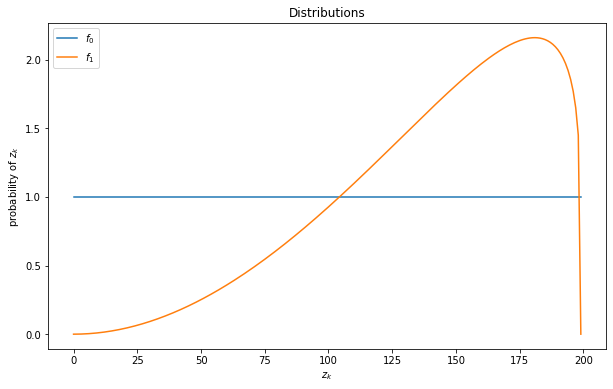### Value Function¶

To solve the model, we will call our solve_model function

In :
h_star = solve_model(wf)    # Solve the model

<ipython-input-7-0c1f615d23a7>:15: NumbaWarning: The TBB threading layer requires TBB version 2019.5 or later i.e., TBB_INTERFACE_VERSION >= 11005. Found TBB_INTERFACE_VERSION = 11004. The TBB threading layer is disabled.
h_new = Q(h, wf)


We will also set up a function to compute the cutoffs $\alpha$ and $\beta$ and plot these on our value function plot

In :
@jit(nopython=True)
def find_cutoff_rule(wf, h):

"""
This function takes a continuation value function and returns the
corresponding cutoffs of where you transition between continuing and
choosing a specific model
"""

π_grid = wf.π_grid
L0, L1 = wf.L0, wf.L1

# Evaluate cost at all points on grid for choosing a model
payoff_f0 = (1 - π_grid) * L0
payoff_f1 = π_grid * L1

# The cutoff points can be found by differencing these costs with
# The Bellman equation (J is always less than or equal to p_c_i)
β = π_grid[np.searchsorted(
payoff_f1 - np.minimum(h, payoff_f0),
1e-10)
- 1]
α = π_grid[np.searchsorted(
np.minimum(h, payoff_f1) - payoff_f0,
1e-10)
- 1]

return (β, α)

β, α = find_cutoff_rule(wf, h_star)
cost_L0 = (1 - wf.π_grid) * wf.L0
cost_L1 = wf.π_grid * wf.L1

fig, ax = plt.subplots(figsize=(10, 6))

ax.plot(wf.π_grid, h_star, label='continuation value')
ax.plot(wf.π_grid, cost_L1, label='choose f1')
ax.plot(wf.π_grid, cost_L0, label='choose f0')
ax.plot(wf.π_grid,
np.amin(np.column_stack([h_star, cost_L0, cost_L1]),axis=1),
lw=15, alpha=0.1, color='b', label='minimum cost')

ax.annotate(r"$\beta$", xy=(β + 0.01, 0.5), fontsize=14)
ax.annotate(r"$\alpha$", xy=(α + 0.01, 0.5), fontsize=14)

plt.vlines(β, 0, β * wf.L0, linestyle="--")
plt.vlines(α, 0, (1 - α) * wf.L1, linestyle="--")

ax.set(xlim=(0, 1), ylim=(0, 0.5 * max(wf.L0, wf.L1)), ylabel="cost",
xlabel="$\pi$", title="Value function")

plt.legend(borderpad=1.1)
plt.show()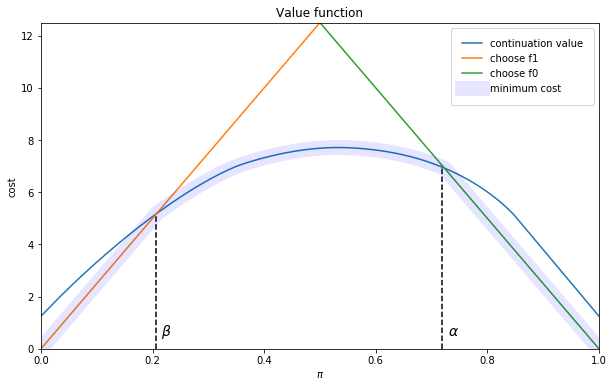The value function equals $\pi L_1$ for $\pi \leq \beta$, and $(1-\pi )L_0$ for $\pi \geq \alpha$.

The slopes of the two linear pieces of the value function are determined by $L_1$ and $- L_0$.

The value function is smooth in the interior region, where the posterior probability assigned to $f_0$ is in the indecisive region $\pi \in (\beta, \alpha)$.

The decision-maker continues to sample until the probability that he attaches to model $f_0$ falls below $\beta$ or above $\alpha$.

### Simulations¶

The next figure shows the outcomes of 500 simulations of the decision process.

On the left is a histogram of the stopping times, which equal the number of draws of $z_k$ required to make a decision.

The average number of draws is around 6.6.

On the right is the fraction of correct decisions at the stopping time.

In this case, the decision-maker is correct 80% of the time

In :
def simulate(wf, true_dist, h_star, π_0=0.5):

"""
This function takes an initial condition and simulates until it
stops (when a decision is made)
"""

f0, f1 = wf.f0, wf.f1
f0_rvs, f1_rvs = wf.f0_rvs, wf.f1_rvs
π_grid = wf.π_grid
κ = wf.κ

if true_dist == "f0":
f, f_rvs = wf.f0, wf.f0_rvs
elif true_dist == "f1":
f, f_rvs = wf.f1, wf.f1_rvs

# Find cutoffs
β, α = find_cutoff_rule(wf, h_star)

# Initialize a couple of useful variables
decision_made = False
π = π_0
t = 0

while decision_made is False:
# Maybe should specify which distribution is correct one so that
# the draws come from the "right" distribution
z = f_rvs()
t = t + 1
π = κ(z, π)
if π < β:
decision_made = True
decision = 1
elif π > α:
decision_made = True
decision = 0

if true_dist == "f0":
if decision == 0:
correct = True
else:
correct = False

elif true_dist == "f1":
if decision == 1:
correct = True
else:
correct = False

return correct, π, t

def stopping_dist(wf, h_star, ndraws=250, true_dist="f0"):

"""
Simulates repeatedly to get distributions of time needed to make a
decision and how often they are correct
"""

tdist = np.empty(ndraws, int)
cdist = np.empty(ndraws, bool)

for i in range(ndraws):
correct, π, t = simulate(wf, true_dist, h_star)
tdist[i] = t
cdist[i] = correct

return cdist, tdist

def simulation_plot(wf):
h_star = solve_model(wf)
ndraws = 500
cdist, tdist = stopping_dist(wf, h_star, ndraws)

fig, ax = plt.subplots(1, 2, figsize=(16, 5))

ax.hist(tdist, bins=np.max(tdist))
ax.set_title(f"Stopping times over {ndraws} replications")
ax.set(xlabel="time", ylabel="number of stops")
ax.annotate(f"mean = {np.mean(tdist)}", xy=(max(tdist) / 2,
max(np.histogram(tdist, bins=max(tdist))) / 2))

ax.hist(cdist.astype(int), bins=2)
ax.set_title(f"Correct decisions over {ndraws} replications")
ax.annotate(f"% correct = {np.mean(cdist)}",
xy=(0.05, ndraws / 2))

plt.show()

simulation_plot(wf)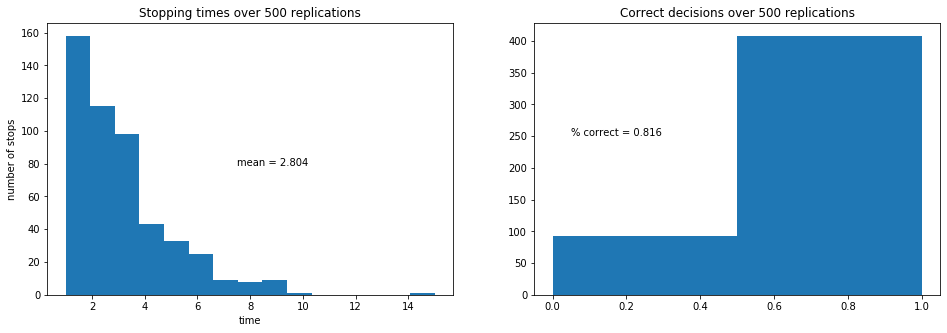### Comparative Statics¶

Now let’s consider the following exercise.

We double the cost of drawing an additional observation.

Before you look, think about what will happen:

• Will the decision-maker be correct more or less often?
• Will he make decisions sooner or later?
In :
wf = WaldFriedman(c=2.5)
simulation_plot(wf)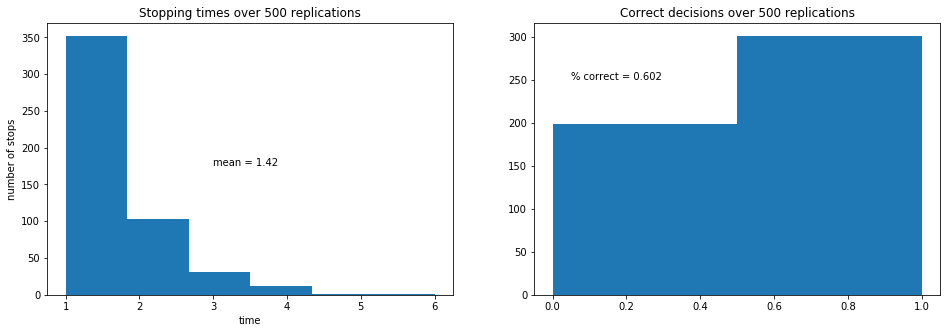Increased cost per draw has induced the decision-maker to take fewer draws before deciding.

Because he decides with fewer draws, the percentage of time he is correct drops.

This leads to him having a higher expected loss when he puts equal weight on both models.

### A Notebook Implementation¶

To facilitate comparative statics, we provide a Jupyter notebook that generates the same plots, but with sliders.

With these sliders, you can adjust parameters and immediately observe

• effects on the smoothness of the value function in the indecisive middle range as we increase the number of grid points in the piecewise linear approximation.
• effects of different settings for the cost parameters $L_0, L_1, c$, the parameters of two beta distributions $f_0$ and $f_1$, and the number of points and linear functions $m$ to use in the piece-wise continuous approximation to the value function.
• various simulations from $f_0$ and associated distributions of waiting times to making a decision.
• associated histograms of correct and incorrect decisions.

## Comparison with Neyman-Pearson Formulation¶

For several reasons, it is useful to describe the theory underlying the test that Navy Captain G. S. Schuyler had been told to use and that led him to approach Milton Friedman and Allan Wallis to convey his conjecture that superior practical procedures existed.

Evidently, the Navy had told Captail Schuyler to use what it knew to be a state-of-the-art Neyman-Pearson test.

We’ll rely on Abraham Wald’s [Wal47] elegant summary of Neyman-Pearson theory.

For our purposes, watch for there features of the setup:

• the assumption of a fixed sample size $n$
• the application of laws of large numbers, conditioned on alternative probability models, to interpret the probabilities $\alpha$ and $\beta$ defined in the Neyman-Pearson theory

Recall that in the sequential analytic formulation above, that

• The sample size $n$ is not fixed but rather an object to be chosen; technically $n$ is a random variable.
• The parameters $\beta$ and $\alpha$ characterize cut-off rules used to determine $n$ as a random variable.
• Laws of large numbers make no appearances in the sequential construction.

In chapter 1 of Sequential Analysis [Wal47] Abraham Wald summarizes the Neyman-Pearson approach to hypothesis testing.

Wald frames the problem as making a decision about a probability distribution that is partially known.

(You have to assume that something is already known in order to state a well-posed problem – usually, something means a lot)

By limiting what is unknown, Wald uses the following simple structure to illustrate the main ideas:

• A decision-maker wants to decide which of two distributions $f_0$, $f_1$ govern an IID random variable $z$.
• The null hypothesis $H_0$ is the statement that $f_0$ governs the data.
• The alternative hypothesis $H_1$ is the statement that $f_1$ governs the data.
• The problem is to devise and analyze a test of hypothesis $H_0$ against the alternative hypothesis $H_1$ on the basis of a sample of a fixed number $n$ independent observations $z_1, z_2, \ldots, z_n$ of the random variable $z$.

To quote Abraham Wald,

A test procedure leading to the acceptance or rejection of the [null] hypothesis in question is simply a rule specifying, for each possible sample of size $n$, whether the [null] hypothesis should be accepted or rejected on the basis of the sample. This may also be expressed as follows: A test procedure is simply a subdivision of the totality of all possible samples of size $n$ into two mutually exclusive parts, say part 1 and part 2, together with the application of the rule that the [null] hypothesis be accepted if the observed sample is contained in part 2. Part 1 is also called the critical region. Since part 2 is the totality of all samples of size $n$ which are not included in part 1, part 2 is uniquely determined by part 1. Thus, choosing a test procedure is equivalent to determining a critical region.

Let’s listen to Wald longer:

As a basis for choosing among critical regions the following considerations have been advanced by Neyman and Pearson: In accepting or rejecting $H_0$ we may commit errors of two kinds. We commit an error of the first kind if we reject $H_0$ when it is true; we commit an error of the second kind if we accept $H_0$ when $H_1$ is true. After a particular critical region $W$ has been chosen, the probability of committing an error of the first kind, as well as the probability of committing an error of the second kind is uniquely determined. The probability of committing an error of the first kind is equal to the probability, determined by the assumption that $H_0$ is true, that the observed sample will be included in the critical region $W$. The probability of committing an error of the second kind is equal to the probability, determined on the assumption that $H_1$ is true, that the probability will fall outside the critical region $W$. For any given critical region $W$ we shall denote the probability of an error of the first kind by $\alpha$ and the probability of an error of the second kind by $\beta$.

Let’s listen carefully to how Wald applies law of large numbers to interpret $\alpha$ and $\beta$:

The probabilities $\alpha$ and $\beta$ have the following important practical interpretation: Suppose that we draw a large number of samples of size $n$. Let $M$ be the number of such samples drawn. Suppose that for each of these $M$ samples we reject $H_0$ if the sample is included in $W$ and accept $H_0$ if the sample lies outside $W$. In this way we make $M$ statements of rejection or acceptance. Some of these statements will in general be wrong. If $H_0$ is true and if $M$ is large, the probability is nearly $1$ (i.e., it is practically certain) that the proportion of wrong statements (i.e., the number of wrong statements divided by $M$) will be approximately $\alpha$. If $H_1$ is true, the probability is nearly $1$ that the proportion of wrong statements will be approximately $\beta$. Thus, we can say that in the long run [ here Wald applies law of large numbers by driving $M \rightarrow \infty$ (our comment, not Wald’s) ] the proportion of wrong statements will be $\alpha$ if $H_0$is true and $\beta$ if $H_1$ is true.

The quantity $\alpha$ is called the size of the critical region, and the quantity $1-\beta$ is called the power of the critical region.

Wald notes that

one critical region $W$ is more desirable than another if it has smaller values of $\alpha$ and $\beta$. Although either $\alpha$ or $\beta$ can be made arbitrarily small by a proper choice of the critical region $W$, it is possible to make both $\alpha$ and $\beta$ arbitrarily small for a fixed value of $n$, i.e., a fixed sample size.

Wald summarizes Neyman and Pearson’s setup as follows:

Neyman and Pearson show that a region consisting of all samples $(z_1, z_2, \ldots, z_n)$ which satisfy the inequality

$$\frac{ f_1(z_1) \cdots f_1(z_n)}{f_0(z_1) \cdots f_0(z_n)} \geq k$$

is a most powerful critical region for testing the hypothesis $H_0$ against the alternative hypothesis $H_1$. The term $k$ on the right side is a constant chosen so that the region will have the required size $\alpha$.

Wald goes on to discuss Neyman and Pearson’s concept of uniformly most powerful test.

Here is how Wald introduces the notion of a sequential test

A rule is given for making one of the following three decisions at any stage of the experiment (at the m th trial for each integral value of m ): (1) to accept the hypothesis H , (2) to reject the hypothesis H , (3) to continue the experiment by making an additional observation. Thus, such a test procedure is carried out sequentially. On the basis of the first observation, one of the aforementioned decision is made. If the first or second decision is made, the process is terminated. If the third decision is made, a second trial is performed. Again, on the basis of the first two observations, one of the three decision is made. If the third decision is made, a third trial is performed, and so on. The process is continued until either the first or the second decisions is made. The number n of observations required by such a test procedure is a random variable, since the value of n depends on the outcome of the observations.

Footnotes

 The decision maker acts as if he believes that the sequence of random variables $[z_{0}, z_{1}, \ldots]$ is exchangeable. See Exchangeability and Bayesian Updating and [Kre88] chapter 11, for discussions of exchangeability.

## Sequels¶

We’ll dig deeper into some of the ideas used here in the following lectures:

• this lecture discusses the key concept of exchangeability that rationalizes statistical learning
• this lecture describes likelihood ratio processes and their role in frequentist and Bayesian statistical theories
• this lecture discusses the role of likelihood ratio processes in Bayesian learning
• this lecture returns to the subject of this lecture and studies whether the Captain’s hunch that the (frequentist) decision rule that the Navy had ordered him to use can be expected to be better or worse than the rule sequential rule that Abraham Wald designed
• Share page